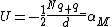Also found in: Wikipedia.

A numerical constant αM in terms of which the electrostatic energy U of a three-dimensional periodic crystal lattice of positive and negativepoint charges q+, -q-, N in number, is given by the equation below, where d is the nearest-neighbor distance between positive and negative charges and N is large. Knowledge of such electrostatic energies as given by the Madelung constant is of importance in the calculation of the cohesive energies of ionic crystals and in many other problems in the physics of solids. See Ionic crystals

Madelung constants for some common ionic crystals
Crystal structure constant, αM
Sodium chloride, NaCl 1.7476
Cesium chloride, CsCI 1.7627
Zinc blende, α-ZnS 1.6381
Wurtzite, β-ZnS 1.641
Fluorite, CaF2 5.0388
Cuprite, CuO2 4.1155
Rutile, TiO2 4.816
Anatase, TiO2 4.800
Corundum, Al2O3 25.0312

The Madelung constants for a number of common ionic crystal structures are given in the table. For these cases d is chosen as the nearest-neighbor distance. See Crystal

McGraw-Hill Concise Encyclopedia of Physics. © 2002 by The McGraw-Hill Companies, Inc.